#### Regret

It is important to note that the models presented here are not the only accepted ways to make good decisions. In game theory, the key idea is to minimize regret.'' This is the feeling you get after making a bad decision and wishing that you could change it after the game is finished. Suppose that after you choose some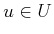, you are told which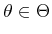was applied by nature. The regret is the amount of cost that you could have saved by picking a different action, given the nature action that was applied.

For each combination ofand, the regret,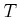, is defined as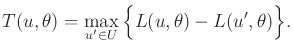(9.20)

For Formulation 9.3, if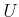and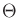are finite, then a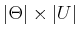regret matrix can be defined.

Suppose that minimizing regret is the primary concern, as opposed to the actual cost received. Under the nondeterministic model, the action that minimizes the worst-case regret is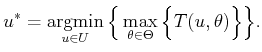(9.21)

In the probabilistic model, the action that minimizes the expected regret is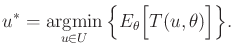(9.22)

The only difference with respect to (9.14) and (9.15) is that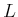has been replaced by. In Section 9.3.2, regret will be discussed in more detail because it forms the basis of optimality concepts in game theory.

Example 9..10 (Regret Matrix)   The regret matrix for Example 9.8 is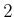00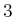00Using the nondeterministic model,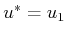, which results in a worst-case regret ofusing (9.21). Under the probabilistic model, let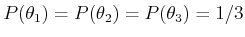. In this case,, which yields the optimal expected regret, calculated asusing (9.22).

Steven M LaValle 2020-08-14